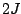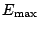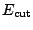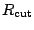Next: Output files Up: Input data file Previous: The namelist input

## The namelist nucleus

This namelist describes the nucleus to be computed, it can be repeated as many times as needed in the input file. Along with the neutron and proton numbers, other quantities can be set, notably the parameters of the Skyrme force in the particle-particle channel. If a variable is not present in the list and has not been present in the previous namelists nucleus, it acquires a default value (see table 4). If a variable is not present but was present in a previous namelist, it keeps its previous value. The variables are

• neutron and proton: number of neutrons and protons for the nucleus. If one of this number is negative then the program will let vary the number of particles in order to reach the corresponding drip line.
• j_max(2): maximum values of(first for neutrons, second for protons). It is reasonable to use different values for neutrons and protons in the case of very asymmetric or semi magic nuclei.
• cut_off and cut_diffuseness: If the regularization procedure is used, the densities are built by summing up contributions from the quasiparticle states up to the maximum equivalent energy (47)given by cut_off, if not, the summation is made up to the cut-off equivalent energy(47) given by cut_off with a smooth Fermi profile of diffuseness cut_diffuseness, see Sec. 2.7.
• r_cut: value of distancebeyond which the paring fields are neglected when integrating the HFB equations, see Eq. (77).
• e_step: initial step in energy (in MeV) for the search of the solution of the HFB equations.
• skt0p and skt3p: parameters of the force in the particle-particle channel as defined in equation (45).
• read_pot: This string can be used to give a name of a file where the initial potentials are read. The number of mesh points can be different in the run and in the saved potentials but the integration step must be the same.
• densities, meanfields, quasiparticle and canonical_states: logical flags which can be set if one wants to save the densities, mean fields and quasiparticle and canonical states wave functions at the end of the run.

Table 4: Variables from the namelist nucleus. The neutron and proton numbers have no default values. If the energy step is not present in the list it is calculated as a function of the box radius. By default, the values of skt0p and skt3p are fixed by the kind of pairing force chosen in the namelist input.
 Variable type Default value neutron integer - proton integer - j_max(2) integer (/21,21/) cut_off real 60.0 cut_diffuseness real 1.0 r_cut real 30.0 e_step real calculated skt0p real predefined skt3p real predefined read_pot character*40 '' densities logical F meanfields logical F quasiparticles logical F canonical_states logical FNext: Output files Up: Input data file Previous: The namelist input
Jacek Dobaczewski 2005-01-23